Next: Colour Texture Boundary Information Up: Image Segmentation Strategy Previous: Initialisation: Perceptual Edges

## Colour Texture Region Information

Colour in a textured region is by definition not homogenous and presents a very variable behaviour through different image regions. Hence, methods which implicitly assume the same shape for all the clusters in the space, are not able to handle the complexity of the real feature space . Therefore, we focus our attention on density estimation from a non-parametric approach since these methods do not have embedded assumptions, and specifically we adopt the kernel estimation technique. Considering colour pixels inside seeds as a set of data points assumed to be a sample of region colour, density estimation techniques allow the construction of an estimate of the probability density function which describes the behaviour of colour in a region. Given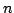data points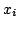,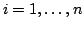in the d-dimensional space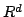, the multivariate kernel density estimator with kernel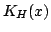and a bandwidth parameter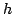, becomes the expression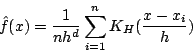(1)

which gives us the probability of a pixel to belong to a region considering colour properties,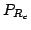, on the three-dimensional colour space. Note that in order to use only one bandwidth parameter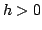the metric of the feature space has to be Euclidean.

On the other hand, texture of each region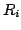is modeled by a multivariate Gaussian distribution considering the set of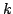texture features extracted from the luminance image. Thus, the mean vector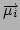and the covariance matrix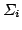, which are initialised from the seeds, describe the texture homogeneity region behaviour. Therefore, the probability of a pixel of belonging to a region taking textural properties into account,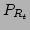, is given by the probability density function of a multivariate Gaussian distribution.

Considering both properties together, colour and texture, the probability of a pixel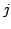of belonging to a regionwill be obtained considering the similarity of the colour pixel with the colour of the region, and the similarity of the texture around the pixel with the texture of the region. The combination of both terms gives the equation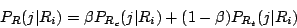(2)

where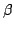weights the relative importance of colour and texture terms to evaluate the region information.Next: Colour Texture Boundary Information Up: Image Segmentation Strategy Previous: Initialisation: Perceptual Edges